Get inspired by the success stories of our students in IIT JAM MS, ISI  MStat, CMI MSc DS.  Learn More

# Telescopic Continuity | ISI MStat 2015 PSB Problem 1This problem is a simple application of the sequential definition of continuity from ISI MStat 2015 PSB Problem 1 based on Telescopic Continuity.

## Problem- Telescopic Continuity

Let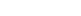be a function which is continuous at 0 and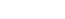Also assume that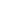satisfies the following relation for all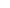: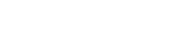Find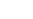.

## Solution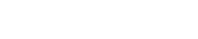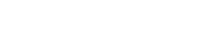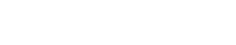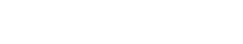Add them all up. That's the telescopic elegance.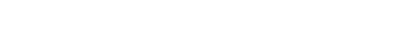Observe that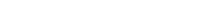since,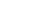is continuous at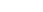.

Hence take limit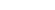on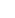, and we get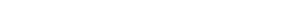.

## Food for Thought

• Find the general function from the given condition.
•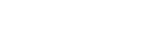and g(x) is continuous, then prove that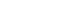.
• What if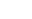is not continuous?

This problem is a simple application of the sequential definition of continuity from ISI MStat 2015 PSB Problem 1 based on Telescopic Continuity.

## Problem- Telescopic Continuity

Letbe a function which is continuous at 0 andAlso assume thatsatisfies the following relation for all:Find.

## SolutionAdd them all up. That's the telescopic elegance.Observe thatsince,is continuous at.

Hence take limiton, and we get.

## Food for Thought

• Find the general function from the given condition.
•and g(x) is continuous, then prove that.
• What ifis not continuous?

This site uses Akismet to reduce spam. Learn how your comment data is processed.

### Knowledge Partner